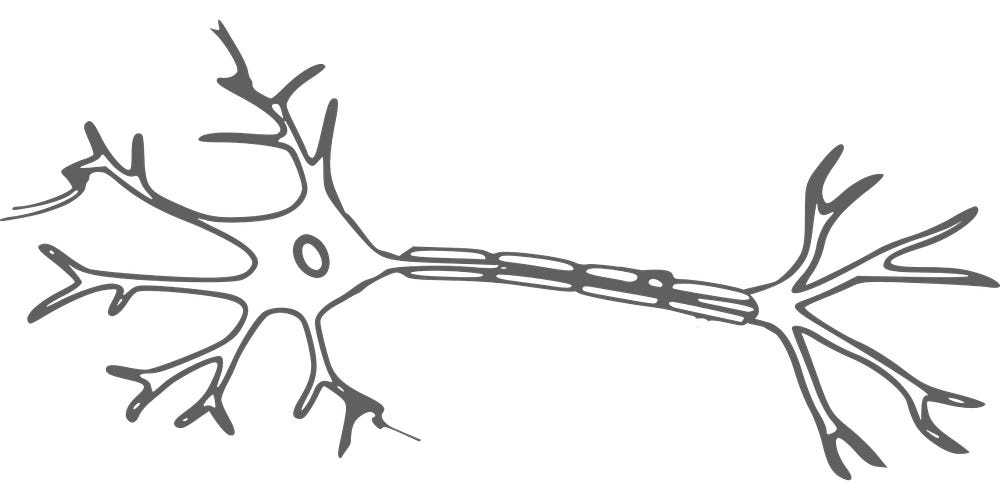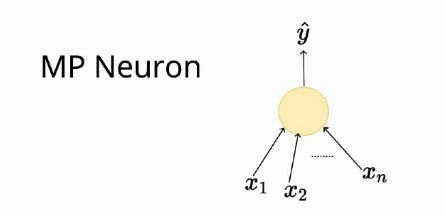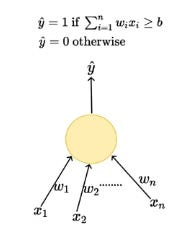# McCulloch-Pitts Neuron vs Perceptron model

Source: Deep Learning on Medium

McCulloch-Pitts Neuron abbreviated as MP Neuron is the fundamental building block of Artificial Neural Network. Similar to biological neurons, both MP Neuron as well as the Perceptron Model take inputs and process them to give an output, although they differ in how they process which we will see in this post down below.

Biological Neuron

But first, we will see how a biological neuron works. Firstly, a neuron can be divided into three basic units, Dendrites, Cell body(also called Soma) and Axon. For every task we do, in our brain, a specific network of these neurons fire signals which pass through Synaptic terminals to Dendrites through the Cell body to Axon which further get transferred to another neuron.A Biological Neuron

McCulloch-Pitts Neuron Model

Proposed by Warren McCulloch and Walter Pitts in 1943, this model imitates the functionality of a biological neuron, thus is also called Artificial Neuron. An artificial neuron accepts binary inputs and produces a binary output based on a certain threshold value which can be adjusted. This can be mainly used for classification problems.An Artificial Neuron ( looks similar to biological neuron, right? )

On taking various inputs the function aggregates them and takes decision based on the aggregation. Aggregation simply means sum of these binary inputs. If the aggregated value exceeds the threshold, the output is 1 else it is 0.

Perceptron Model

This model was developed by Frank Rosenblatt in 1957. This is a slightly tweaked version of the Artificial Neuron model we saw earlier. Here, the neurons are also called Linear Threshold Unit (LTU). This model can work on non-boolean values where each input connection gets associated with a weight. Here the function calculates the weighted sum and based on the threshold value provided, it gives a binary output.As seen in the image above, every input connection in the Perceptron is associated with a weight which determines how much that input affects the output y hat.

Comparison between MP Neuron Model and Perceptron Model

• Both, MP Neuron Model as well as the Perceptron model work on linearly separable data.
• MP Neuron Model only accepts boolean input whereas Perceptron Model can process any real input.
• Inputs aren’t weighted in MP Neuron Model, which makes this model less flexible. On the other hand, Perceptron model can take weights with respective to inputs provided.
• While using both the models we can adjust threshold input to make the model fit our the dataset.

You can find the paper McCulloch and Pitts published, here .

This is the first post from the series on Deep learning I would be posting over a span of five months. So, stay tuned. :)